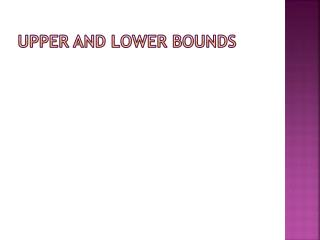# Upper and lower bounds - PowerPoint PPT PresentationDownload PresentationUpper and lower bounds

Upper and lower boundsDownload Presentation## Upper and lower bounds

- - - - - - - - - - - - - - - - - - - - - - - - - - - E N D - - - - - - - - - - - - - - - - - - - - - - - - - - -
##### Presentation Transcript

1. Upper and lower bounds

2. Upper and Lower Bounds Objectives • All can work out Upper and Lower Bounds of discrete data. • Most can apply Upper and Lower Bounds of continuous data. • Some students can extend what they have learnt to harder scenarios.

3. Key words • Upper Bound • Lower Bound • Discrete Data • Continuous Data • Rounding • Greater than / Less than • Inequality

4. Discrete and continuous data Numerical data can be discrete or continuous. Discretedata can only take certain values. For example, shoe sizes, the number of children in a class, amounts of money Continuous data comes from measuring and can take any value within a given range. For example, the weight of a banana, the time it takes for pupils to get to school, heights of 15 year-olds.

5. Upper and lower bounds for discrete data The population of the United Kingdom is 59 million to the nearest million. What is the least this number could be? The least this number could be before being rounded up is: 58 500 000 What is the most this number could be? The most this number could be before being rounded down is: 59 499 999

6. Upper and lower bounds We can give the possible range for the population as: 58 500 000 ≤ population ≤59 499 999 This value is called the lower bound… … and this value is called the upper bound. This is an inequality. It says that the actual population of the United Kingdom is between 58 500 000 and 59 499 999.

7. Upper and lower bounds for discrete data

8. Upper and Lower Bounds Objectives • All can work out Upper and Lower Bounds of discrete data. • Most can apply Upper and Lower Bounds of continuous data. • Some students can extend what they have learnt to harder scenarios.

9. Upper and lower bounds for continuous data The height of the Eiffel Tower is 324 metres to the nearest metre. What is the least this measurement could be? The least this measurement could be before being rounded up is: 323.5 m What is the most this measurement could be? The most this measurement could be before being rounded down is up to but not including: 324.5 m

10. Upper and lower bounds We can write the range for this measurement as: 323.5 m ≤ height <324.5 m This value is called the lower bound … … and this value is called the upper bound. Key Point…..

11. Upper and lower bounds

12. Upper and Lower Bounds Objectives • All can work out Upper and Lower Bounds of discrete data. • Most can apply Upper and Lower Bounds of continuous data. • Some students can extend what they have learnt to harder scenarios.

13. Plenary • A = 5 to the nearest unit • B = 16 to the nearest unit • What are the Upper and Lower Bounds of… • A + B • A X B • A – B 20, 22 69.75, 90.75 10, 12